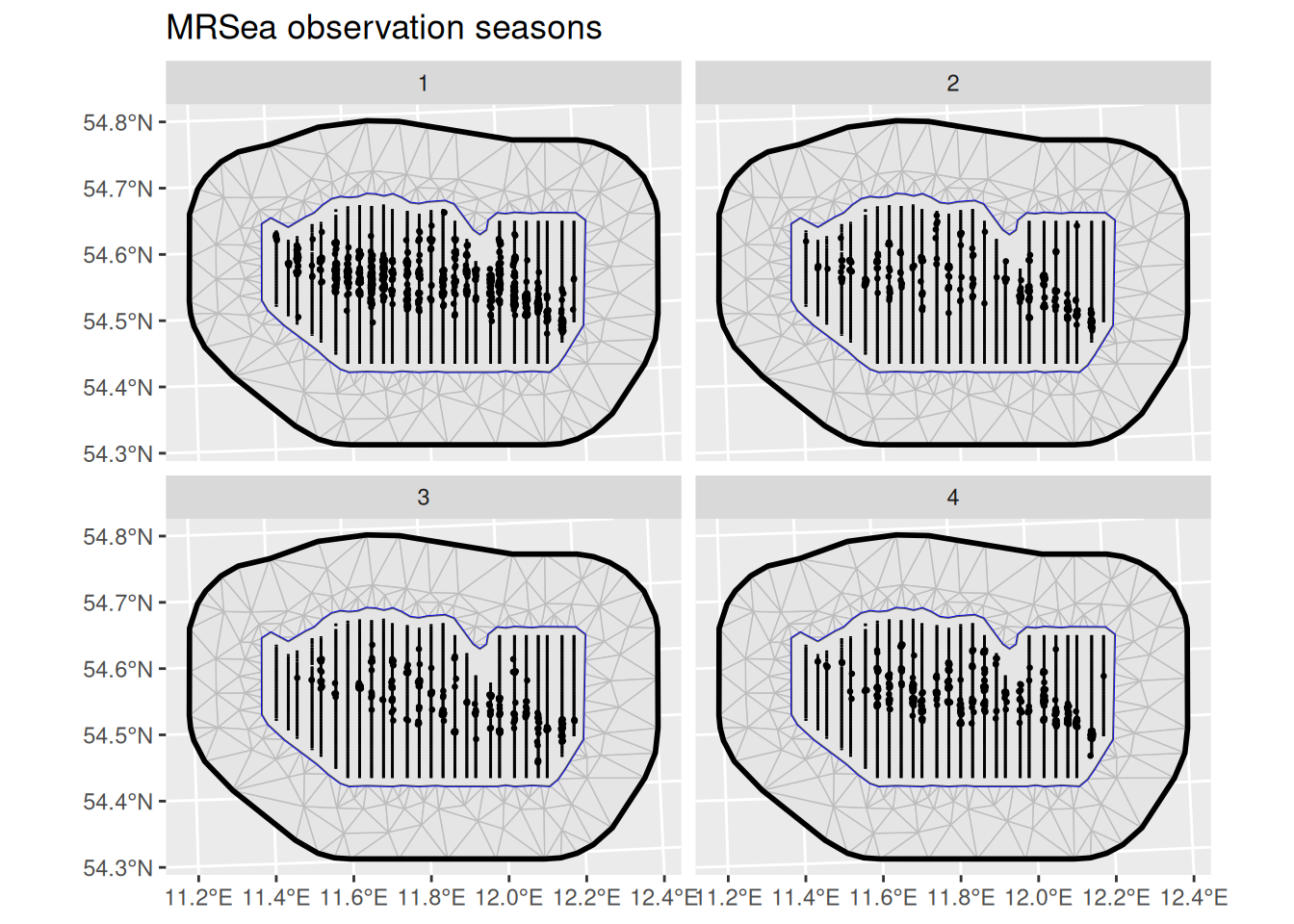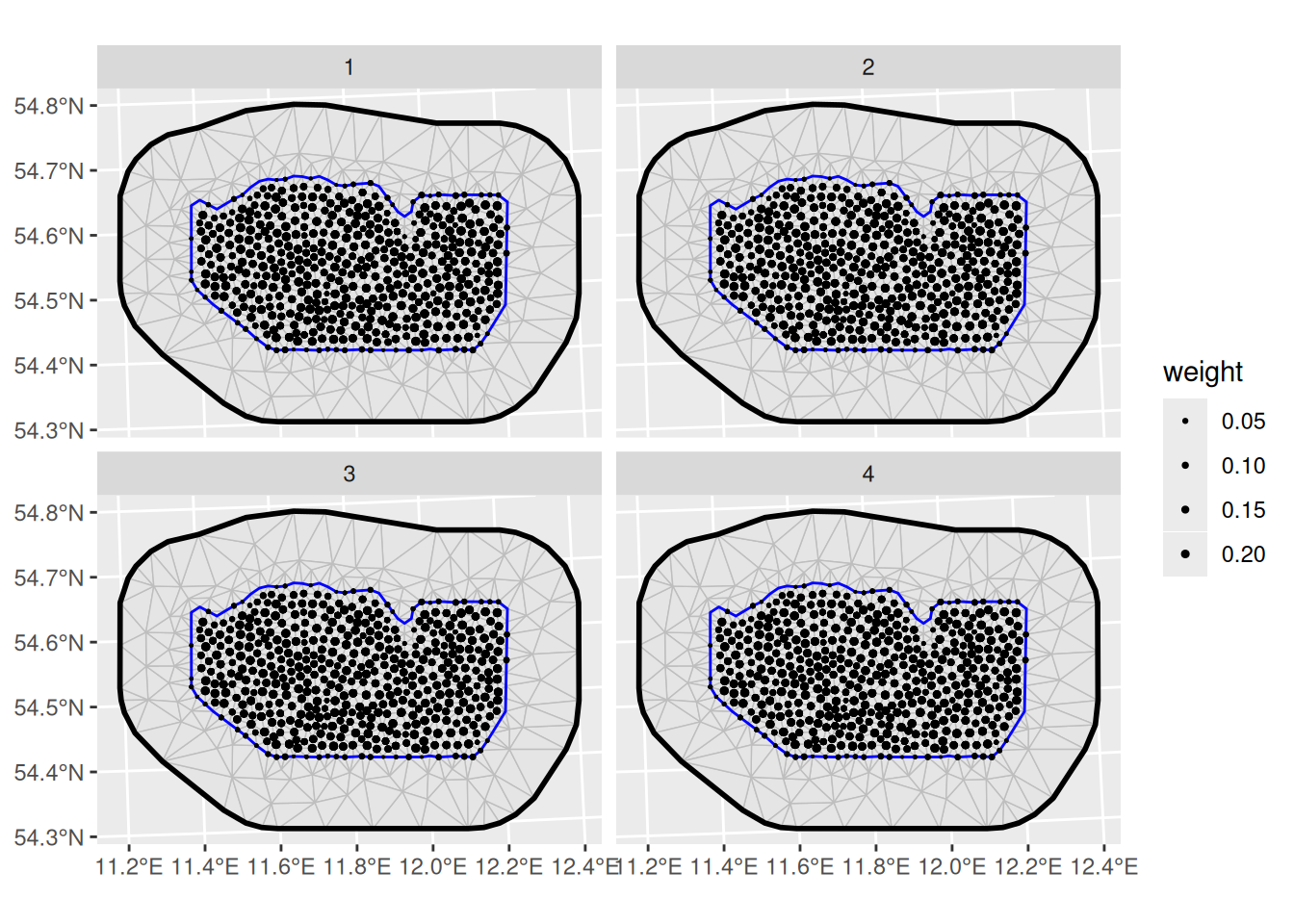## Introduction

For this vignette we are going to be working with a dataset obtained from the R package MRSea. We will set up a LGCP with a spatio-temporal SPDE model to estimate species distribution.

## Setting things up

library(inlabru)
library(INLA)
library(ggplot2)

## Get the data

Load the dataset, that has coordinates in UTM in kilometres:

data(mrsea, package = "inlabru")

The points (representing animals) and the sampling regions of this dataset are associated with a season. Let’s have a look at the observed points and sampling regions for all seasons:

ggplot() +
gg(mrsea$mesh) + gg(mrsea$boundary) +
gg(mrsea$samplers) + gg(mrsea$points, size = 0.5) +
facet_wrap(~season) +
ggtitle("MRSea observation seasons")## Integration points

The inlabru point process model knows how to construct the numerical integration scheme for the LGCP likelihood. We can also call the internal functions directly to see what the integration scheme will look like. Because our model will take time (season) into account we have to construct the integration points for the LGCP accordingly. Using the fm_int() we can specify the product domain space. Note that omitting this step would simply aggregate all sampling regions over time.

ips <- fm_int(
domain = list(coordinates = mrsea$mesh, season = 1:4), samplers = mrsea$samplers
)

Plot the integration points:

ggplot() +
gg(mrsea$mesh) + gg(ips, aes(size = weight)) + scale_size_area(max_size = 1) + facet_wrap(~season)## Fitting the model Fit an LGCP model to the locations of the animals. In this example we will employ a spatio-temporal SPDE. Note how the group and ngroup parameters are employed to let the SPDE model know about the name of the time dimension (season) and the total number of distinct points in time. matern <- inla.spde2.pcmatern(mrsea$mesh,
prior.sigma = c(0.1, 0.01),
prior.range = c(10, 0.01)
)

cmp <- coordinates + season ~ Intercept(1) +
mySmooth(
coordinates,
model = matern,
group = season,
ngroup = 4
)

fit <- lgcp(cmp,
data = mrsea$points, samplers = mrsea$samplers,
domain = list(
coordinates = mrsea$mesh, season = seq_len(4) ) ) Predict and plot the intensity for all seasons: ppxl <- fm_pixels(mrsea$mesh, mask = mrsea$boundary, format = "sp") ppxl_all <- fm_cprod(ppxl, data.frame(season = seq_len(4))) lambda1 <- predict( fit, ppxl_all, ~ data.frame(season = season, lambda = exp(mySmooth + Intercept)) ) pl1 <- ggplot() + gg(as(lambda1, "SpatialPixelsDataFrame"), aes(fill = q0.5)) + gg(mrsea$points, size = 0.3) +
facet_wrap(~season) +
coord_equal()
pl1